Courses

# Test: The D And F-Block Elements 1 - From Past 28 Years Questions

## 35 Questions MCQ Test Chemistry Class 12 | Test: The D And F-Block Elements 1 - From Past 28 Years Questions

Description
This mock test of Test: The D And F-Block Elements 1 - From Past 28 Years Questions for Class 12 helps you for every Class 12 entrance exam. This contains 35 Multiple Choice Questions for Class 12 Test: The D And F-Block Elements 1 - From Past 28 Years Questions (mcq) to study with solutions a complete question bank. The solved questions answers in this Test: The D And F-Block Elements 1 - From Past 28 Years Questions quiz give you a good mix of easy questions and tough questions. Class 12 students definitely take this Test: The D And F-Block Elements 1 - From Past 28 Years Questions exercise for a better result in the exam. You can find other Test: The D And F-Block Elements 1 - From Past 28 Years Questions extra questions, long questions & short questions for Class 12 on EduRev as well by searching above.
QUESTION: 1

### Which of the following statement is not correct? 

Solution:

La (OH)3 is more basic than Li (OH)3. In lanthanides the basic character of hydroxides decreases as the ionic radius decreases.

QUESTION: 2

### General electronic configuration of lanthanides is

Solution:

The Lanthanides are transition metals from Atomic numbers 58 (Ce) to 71(Lu).
Hence the electron configulation becomes : (n –2) f 1– 14 (n – 1) s2p6 d0 – 1 ns2.

QUESTION: 3

### Which of the following shows maximum number of oxidation states? 

Solution:

Mn : [Ar] 3d5  4s2
Shows +2, +3, +4, +5, +6 & +7 oxidation states

QUESTION: 4

The basic character of the transition metal monoxides follows the order  (Atomic Nos.,Ti = 22, V = 23, Cr = 24, Fe = 26)

Solution:

The basic character of the transition metal monoxide is TiO > VO > CrO > FeO because basic character of oxides decrease with increase in atomic number. Oxides of transitional metals in low oxidation state i.e., + 2 and + 3 are generally basic except Cr2O3.

QUESTION: 5

Which one of the following characteristics of the transition metals is associated with their catalytic activity? 

Solution:

Transition metals have the ability to utilize (n –1)d -orbitals. Thus variable oxidation states enables the transition element to associate with the reactants in different forms.

QUESTION: 6

The correct order of ionic radii of Y3+, La3+, Eu3+ and Lu3+ is 

Solution:

In lant hani de ser ies there is a regular decrease in the atomic as well as ionic radii of trivalent ions (M3+) as the atomic number increases. Although the atomic radii do show some irregularities but ionic radii decreases from La(103 pm) to Lu (86pm).

QUESTION: 7

Among the following series of transition metal ions, the one where all metal ions have 3d2 electronic configuration is(At. nos.  Ti = 22; V = 23; Cr = 24; Mn = 25) 

Solution:

The electronic configuration of  different species  given in the question are :

(a)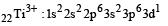(b)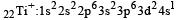(c)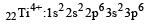(d)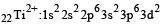Thus options (a) and (c) are discarded; now

let us observe the second point of difference.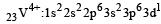Thus option (b) is discarded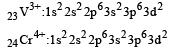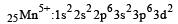QUESTION: 8

Lanthanoids are 

Solution:

Lanthanides are 4 f-series elements starting from cerium  (Z= 58) to lutetium ( Z = 71).
These are placed in the sixth period and in third group.

QUESTION: 9

The aqueous solution containing which one of the following ions will be colourless? (Atomic number: Sc = 21, Fe = 26, Ti = 22, Mn = 25)

Solution: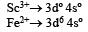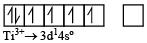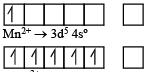In Sc3+ there as no unpaired electron So the aqueous solution of Sc3+ will be colourless.

QUESTION: 10

The main reason for larger number of oxidation states exhibited by the actinoids than the corresponding lanthanoids, is [2005, 2006]

Solution:

The cause of showing different oxidation is due to the fact that there is only a small difference between the energies of electron in the ns orbitals and (n – 1)d orbitals with the result both ns as well (n – 1)d electrons can be used for compound formation. Lesser energy difference between 5f and 6d orbitals than between 4f and 5d orbitals result in larger no. of oxidation state.

QUESTION: 11

Four successive members of the first row transition elements are listed below with their atomic numbers. Which one of them is expected to have the highest third ionization enthalpy? [2 00 5]

Solution:

For third ion ization enthalpy last configuration of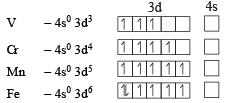For third Ionization enthalpy Mn has stable configuration due to half filled d-orbital.

QUESTION: 12

Copper sulphate dissolves in excess of KCN to give

Solution:

Copper sulphate on treatment with excess of KCN forms complex K3[Cu(CN)4] or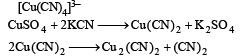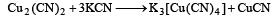QUESTION: 13

In which of the following pairs are both the ions coloured in aqueous solutions ?          (At. no. : Sc = 21, Ti = 22, Ni = 28, Cu = 29, Co = 27)

Solution:

Sc3+ : 1s2, 2s2p6, 3s2p6d0, 4s0; no unpaired electron.
Cu+ : 1s2, 2s2p6, 3s2p6d10, 4s0; no unpaired electron.
Ni2+: 1s2, 2s2p6, 3s2p6d8, 4s0; unpaired electron present.
Ti3+ : 1s2, 2s2p6, 2s2p6d1, 4s0; unpaired electron present Co2+ : 1s2, 2s2p6, 3s2p6d7, 4s0; unpaired electron present So from the given option the only correct combination is Ni2+ and Ti3+

QUESTION: 14

Which of the following oxidation states are the most characteristic for lead and tin respectively?

Solution:

All the group IV elements show tetravalency which can be explained on the basis of shifting of one eof ns to the vacant np orbital.These four orbitals give rise to four sp3 hybrid orbitals.
When ns2 electrons of the outermost shell do not participate in bonding it is called inert pair and the effect is called inert pair effect. The last three elements of group 4 i.e. Ge, Sn and Pb, have a tendency to form divalent as well as tetravalent ions. Since the inert pair effect increases from Ge to Pb, the stability of tetravalent ion decreases and that of divalent ion increases that is why Pb++ is more stable than Pb4+ and Sn4+ ions is more stable than Sn2+.

QUESTION: 15

Which one of the following ions is the most stable in aqueous solution?               (At.No. Ti = 22, V = 23, Cr = 24, Mn = 25)

Solution:

For chromium ion + 3oxidation state is most stable.

QUESTION: 16

Identify the incorrect statement among the following: 

Solution:

There is a steady decrease in the radii as the atomic number of the lanthanide elements increases. For every additional proton added in nucleus the corresponding electron goes to 4f subshell.
The shape of f -orbitals is very much diffused and they have poor shielding effect. The effective nuclear charge increases which causes the contraction in the size of electron charge cloud. This contraction in size is quite regular and known as Lanthanoid contraction.
Since the change in the ionic radii in the lanthanide series is very small, thus their chemical properties are similar.

QUESTION: 17

The correctorder of decreasing second ionisation enthalpy of Ti (22), V(23), Cr(24) and Mn (25) is : 

Solution:

Ti ; Z (22) is 1s22s22p63s23p64s23d2
V ; Z (23) is 1s22s22p63s23p64s23d3
Cr ; Z (24) is 1s22s22p63s23p63d54s1
Mn ; Z (25) is 1s22s22p63s23d54s2

The second electron in all the cases (except Cr) is taken out from 4s-orbital and for Cr it is an electron from completely half filled 3d-orbital. The force required for removal of second electron will be more for Mn (except for Cr) having more positive charge.
Based on this we find the correct order is Mn > V > Ti.  Cr > Mn > V > Ti. So correct answeris (a).

QUESTION: 18

Which one of the elements with the following outer orbital configurations may exhibit the largest number of oxidation states? 

Solution: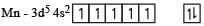The no. of various oxidation states possible are +  2, + 3, + 4, (+ 5), + 6, + 7.

QUESTION: 19

Qut of TiF62–, CoF63–, Cu2Cl2 and  NiCl24– (Z of Ti = 22, Co = 27, Cu = 29, Ni = 28), the colourless species are: 

Solution:

The colour exhibited by transition metal ions is due to the presence of unpaired electrons in -orbitals which permits the  - excitation of electrons.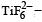Ti is in + 4 O.S. ; 3d0 = colourless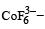Co is in + 3 O.S ; 3d5 = coloured

In Cu2Cl2– Cu is in +1 O.S. ; 3d10 – colourless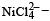Ni is in + 2 O.S ; 3d8 – coloured

QUESTION: 20

Which of the following ions will exhibit colour in aqueous solutions? 

Solution: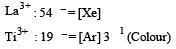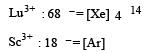QUESTION: 21

Which one of the following ions has electronic configuration [Ar] 3d6 ? 

(At. Nos. Mn = 25, Fe = 26, Co = 27, Ni = 28)

Solution: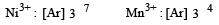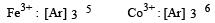QUESTION: 22

Which of the following pairs has the same size?

Solution:

Due to lanthanide contraction, the size of Zr and Hf (atom and ions) become nearly similar

QUESTION: 23

Which of the following oxidation states is the most common among the lanthanoids? 

Solution:

Correct choice : (a)

QUESTION: 24

For the four successive transition elements (Cr, Mn, Fe and Co), the stability of +2 oxidation state will be there in which of the following order?

Solution:
QUESTION: 25

Acidified K2Cr2O7 solution turns green when Na2SO3 is added to it. This is due to the formation of : 

Solution:

The green colour appears due to the formation of Cr+++ion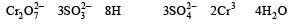QUESTION: 26

Which of the statements is not true? 

Solution:

Na2Cr2O7 is hygroscopic.

QUESTION: 27

Which one of the following does not correctly represent the correct order of the property indicated against it? [2012 M]

Solution:

The melting points of the transition element first rise to a maximum and then fall as the atomic number increases manganese have abnormally low melting points.

QUESTION: 28

Four successive members of the first series of the transition metals are listed below. For which one of them the standard potential (E0M2+ /M) value has a positive sign? [2012 M]

Solution: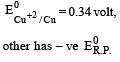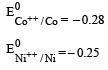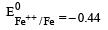QUESTION: 29

The catalytic activity of transition metals and their compounds is ascribed mainly to :[2012 M]

Solution:

The transition metals and their compounds are used as catalysts. Because of the variable oxidation states, due to this, they easily absorb and re-emit wide range of energy to provide the necessary activation energy.

QUESTION: 30

Which of the following exhibit only + 3 oxidation state ? [2012 M]

Solution:

Ac (89) = [Rn] [6 1] [7 2]

QUESTION: 31

Which of the following lanth anoidions is diamagnetic ? (At nos. Ce = 58, Sm = 62, Eu = 63, Yb = 70) [NEET 2013]

Solution:

Sm2+(Z = 62) [Xe]4f6 6s2 – 6 unpaired e
Eu2+(Z = 63) [Xe]4f7 6s2 – 7 unpaired e
Yb2+(Z = 70) [Xe]4f14 6s2 – 0 unpaired e
Ce2+(Z = 58) [Xe]4f1 5d1 6s2 – 2 unpaired e Only Yb2+ is diamagnetic.

QUESTION: 32

KMnO4 can be prepared from K2MnO4 as per the reaction: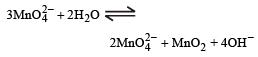The reaction can go to completion by removing OH ions by adding. [NEET 2013]

Solution:

HCl and SO2 are reducing agents and can reduce MnO4. CO2 which is neither oxidising and nor reducing will provide only acidic medium. It can shift reaction in forward direction and reaction can go to completion.

QUESTION: 33

Which of the following statements about the interstitial compounds is incorrect ?[NEET 2013]

Solution:

In interstitial compounds small atoms like H, B & C enter into the void sites between the packed atoms of crystalline metal.
They retain metallic conductivity and are chemically inert.

QUESTION: 34

The outer electronic configuration of Gd (At. No. 64) is: [NEET Kar. 2013]

Solution:

Gd  = [Xe]  4f7 5d1 6s2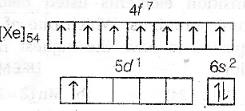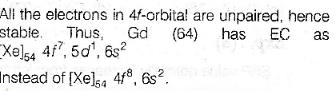QUESTION: 35

Sc (Z = 21) is a transition element but Zn (Z = 30) is not because [NEET Kar. 2013]

Solution:

A transition element must have incomplete -subshell. Zinc have completely filled subshell having 310 configuration. Hence do not show properties of transition elements to any appreciable extent except for their ability to form complexes.